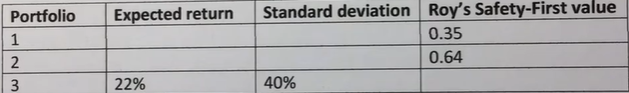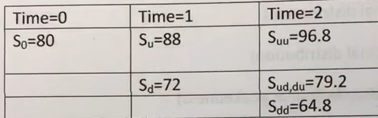## Free CFA Level 1 Test 52 Practice Sample Questions Answer Keys AMBIPi

Hi CFA Aspirants, welcome to AKVTutorials. Are you preparing for CFA Level 1, 2, 3 exams for making a career in CFA (Charted Financial Analyst). According to CFA Wikipedia, CFA The Chartered Financial Analyst (CFA) program is a postgraduate professional certification offered internationally by the American-based CFA Institute. A candidate who successfully completes the program and meets other professional requirements is awarded the “CFA charter” and becomes a “CFA charter holder”. Therefore, you need CFA Study Notes and Free CFA Level 1 Test 52 Practice Sample Questions Answer Keys AMBIPi

In this article, you will get Free CFA Level 1 Mock Exam Practice Questions.

## Free CFA Level 1 Mock Practice Exam Questions Bank

Free CFA Level 1 Practice Question No: 511:

A two-tailed test of the null hypothesis that the mean of a distribution is equal to 4.00 has a p-value of 0.0567. Using a 5% level of significance (i.e., α =0.05), the best conclusion is to:

Option A : reject the null hypothesis

Option B : fail to reject the null hypothesis

Option C : increase the level of significance to 5.67%

Option B : fail to reject the null hypothesis

CFA Level 1 Exam Question No: 512:

In Elliott Wave Theory, Wave 2 commonly exhibits a pattern best described as a(n):

Option A: basing pattern consisting of five smaller waves

Option B: Fibonacci ratio percentage retracement composed of three smaller waves

Option C: uptrend moving above the high of Wave 1 and consisting of five smaller waves

Option B :Fibonacci ratio percentage retracement composed of three smaller waves

Free CFA Level 1 Mock Exam Question No: 513:

An investor wants to maximize the possibility of earning at least 5% on her investments each year. Using Roy’s safety-first criterion, which of the following portfolios is the most appropriate choice?Option A: Portfolio 1

Option B: Portfolio 2

Option C: Portfolio 3

Option B : Portfolio 2

CFA Level 1 Free Practice Question No: 514:

Which of the following is the least likely characteristic of the normal probability distribution? The normal probability distribution:

Option A: has kurtosis of 3.0

Option B: has the same value for mean, median, and mode.

Option C: is more suitable as a model for asset prices than for returns.

Option C : is more suitable as a model for asset prices than for returns.

Free CFA Practice Question No: 515:

The nominal (quoted) annual interest rate on an automobile loan is 10%.The effective annual rate of the loan is 10.47%. The frequency of compounding periods per year for the loan is closest to:

Option A: weekly

Option B: monthly

Option C: quarterly

Option B : monthly

CFA Level 1 Sample Question No: 516:

Equity return series are best described as, for the most part:

Option A: platykurtotic (less peaked than a normal distribution)

Option B: leptokurtotic (more peaked than a normal distribution)

Option C: mesokurtotic (identical to the normal distribution in peakedness)

Option B : leptokurtotic (more peaked than a normal distribution)

Free CFA Level 1 Quiz Question NO: 517:

Event X and event Y are independent events. The probability of X is 0.2 [p(x)=0.2] and the probability of Y is 0.5 [P(Y)=0.5]. The joint probability of X and Y [P(X,Y)] is closest to:

Option A: 0.1

Option B: 0.3

Option C: 0.7

Option A : 0.1

Free CFA Level 1 Quiz Question NO: 518:

A stock is declining in price and reaches a price range wherein buying activity is sufficient to stop the decline. This is best described as a:

Option A: support level

Option B: resistance level

Option C: change in polarity point

Option A : support level

Free CFA Practice Question No: 519:

Assume that a stock’s price over the next two periods is as shown blow.The initial value of the stock is \$80. The probability of an up move in any given period is 75% and the probability of a down move in any given period is 25%. Using the binomial model, the probability that the stock’s price will be \$79.20 at the end of two periods is closest to:

Option A: 18.75%

Option B: 37.5%

Option C: 56.25%

Option B : 37.5%

CFA Mock Exam Free Question No: 520:

Which of the following statements of null and alternative hypotheses requires a two-
tailed test?

Option A: H0: θ = θ0 versus Ha: θ ≠ θ0

Option B: H0: θ ≤ θ0 versus Ha: θ > θ0

Option C: H0: θ ≥ θ0 versus Ha: θ < θ0